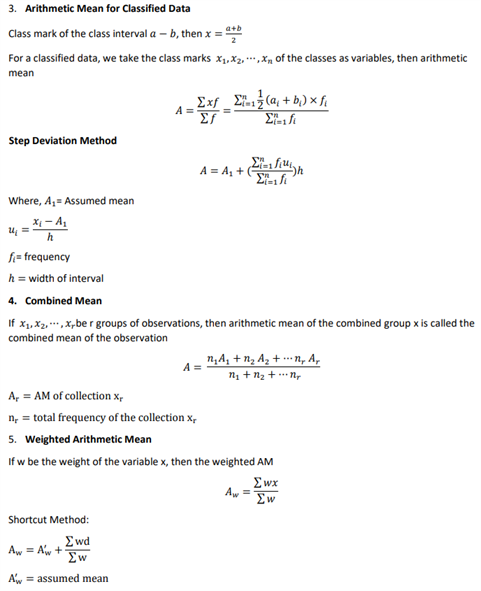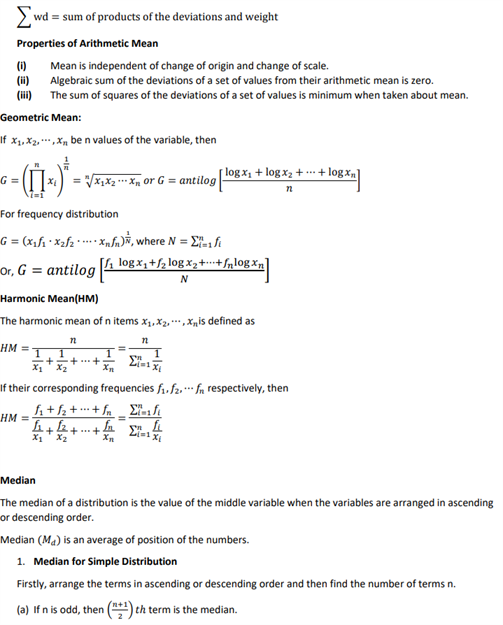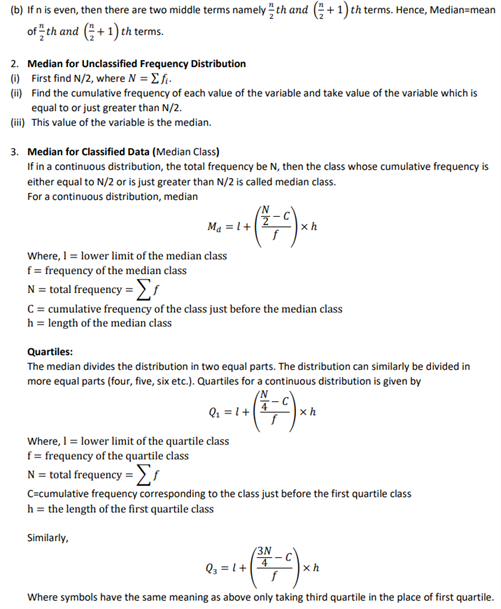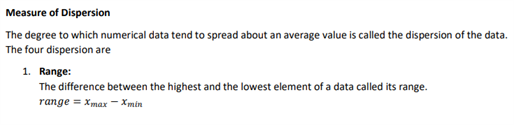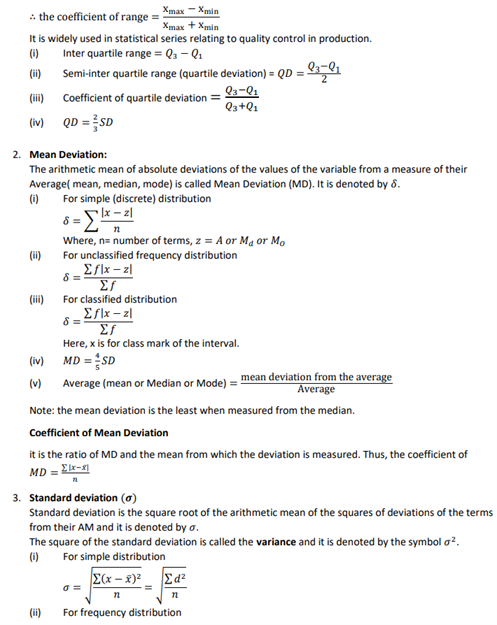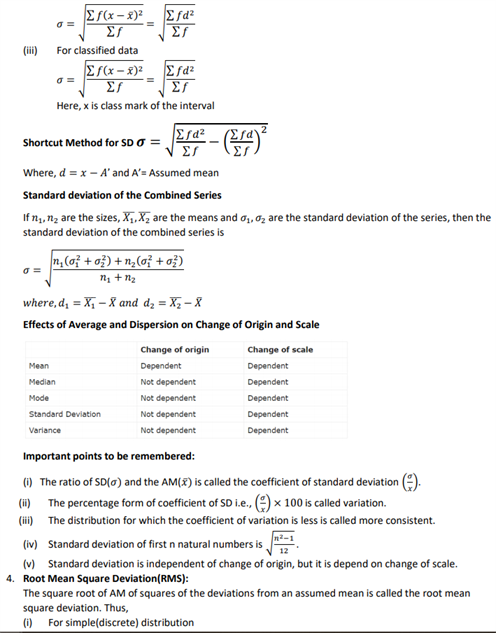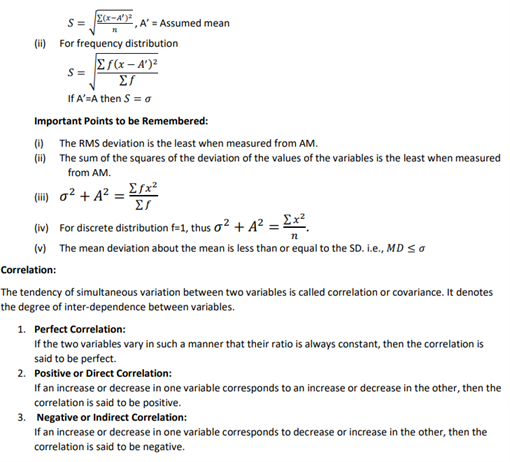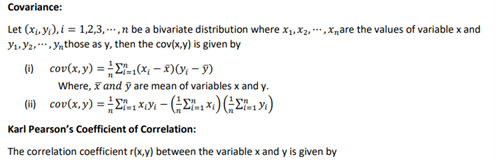# Revision Notes For CBSE Class 11 Maths Chapter 15 Statistics

Students can find the class 11 maths revision notes chapter 15 statistics here. These notes comprise all the explanation with short notes about statistics. They will find how to measure central tendency and along with that, they will find the definition of various terms such as arithmetic mean, combined mean etc. But First, let’s see the definition of various basic terms used in this chapter:

• Limit of the Class:

The starting and end values of each class are called Lower and Upper limit.

• Class Interval:

The difference between upper and lower boundary of a class is called class interval or size of the class.

• Primary and Secondary Data:

The data collected by the investigator himself is known as the primary data, while the data collected by a person, other than the investigator is known as the secondary data.

• Variable or Variate: A characteristics that vary in magnitude from observation to observation. E.g., weight, height, age, etc., are variables.
• Frequency: The number of time an observation occurs in the given data, is called the frequency of the observation.
• Discrete Frequency Distribution: A frequency distribution is called a discrete frequency distribution if the data are presented in such a way that exact measurements of the units are clearly shown.
• Continuous Frequency Distribution: A frequency in which data are arranged in classes groups which are not exactly measurable.

This note provides important relations between mean mode and median, properties of corelation etc. These notes are very important for preparation in exams.

See the Class 11 Revision notes for maths chapter 15 statistics below.